# Multiplying Binomials

 Would You Rather Listen to the Lesson?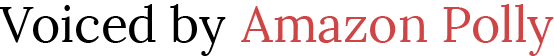Once they achieve mastery in adding and subtracting polynomials, Algebra 1 students go on to learn how to multiply or divide polynomials, with the help of the distributive property. Before learning how to multiply long expressions, students engage in multiplying binomials.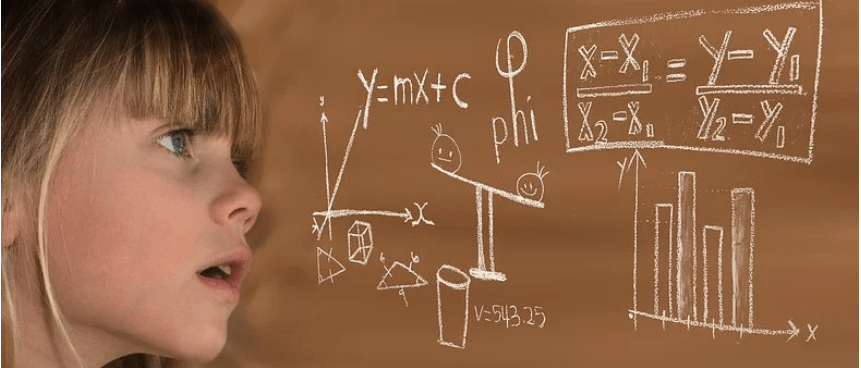## How to Teach Multiplying Binomials

### What Are Binomials?

For starters, you can remind children what binomials are. Binomials are a type of polynomials, representing a sum or difference of two terms, that is, of two monomials. These terms can be a variable, a constant, or an exponent.

Provide a few examples, such as:

3 m² – 7

5 + 3x

13x – 1

### Review the Distributive Property

Since learning how to multiply binomials requires having a solid foundation in the distributive property, it makes sense to review it.

We can present the distributive property of multiplication over addition as:

a x (b + c) = a x b + a x c

And:

(b + c) x a = b x a + c x a

Whereas the distributive property of multiplication over subtraction is presented as:

a x (b – c) = a x b – a x c

And:

(b – c) x a = b x a – c x a

You can also check out our article on teaching the distributive property.

### How to Multiply Binomials

There are several methods that students learn to multiply binomials, such as multiplying binomials vertically, multiplying binomials horizontally, or multiplying binomials using a grid. There is also a special method, called the FOIL method, used only for multiplying one binomial by another binomial.

In this article, we’ll focus on the FOIL method, but you can also refer to these Guided Notes (Members Only) for more instructions on the other methods.

In addition, if you have the possibility, you can also choose to show a few videos at the beginning of the lesson or throughout it in order to help children understand the process of multiplication better.

This video by Khan Academy is a free resource on how to multiply binomials by using the grid or area model, and this video contains step-by-step instructions on using the FOIL method.

#### Multiplying Tiny Expressions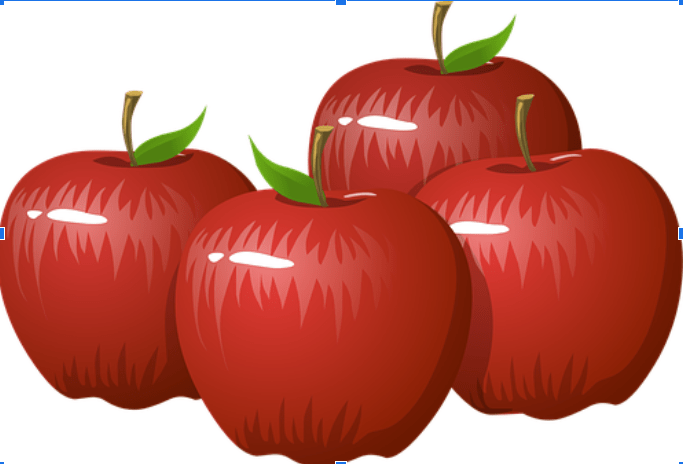First provide an example of multiplication of a tiny expression, such as monomials:

2x multiplied by 3 = 6x

Just like two apples times three is 6 apples!

2x multiplied by 3x = 6x²

So we multiply the x’s as well!

#### Multiplying Binomials: Example 1

So far, so good! But if we look at two binomials and try to multiply them, things get a bit more complicated. Provide an example of two such binomials:

(x + 8) (x – 2) = ?

Explain to students that the FOIL method stands for: First, Outer, Inner, and Last. So we have to multiply in this exact order and the abbreviation FOIL helps us remember the order! Remind students to carry the sign.

You can also illustrate the First, Outer, Inner, and Last order on the whiteboard by using markers in different colors so that it’s easier for children to remember it: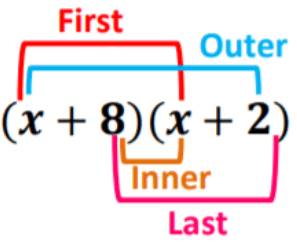In other words, we’ll have:

First: x multiplied by x = x²

Outer: x multiplied by 2 = 2x

Inner: 8 multiplied by x = 8x

Last: 8 multiplied by 2 = 16

By doing this, we are basically distributing each term in one binomial across the other binomial term. That is, we’re using the distributive property twice.

Point out to students that when we multiply a binomial by a binomial, we’ll end up with four terms. It may be possible to create a trinomial by combining similar terms but there will be cases where there aren’t any similar terms, so we’ll just keep the four terms.

So at this point, the only thing left to check if we can combine like terms. You can also use a highlighter to highlight such terms.

(x + 8) (x – 2) = x²+ 2x + 8x + 16

So in this expression, we see that 2x and 8x are like terms and that we can simplify the expression by combining them, or in simple terms, adding them. By doing that, we’ll get the following trinomial:

x² + 10x + 16

#### Multiplying Binomials: Example 2

The above example was an example of two binomials with the same sign (addition) in between the terms of the binomials. But one of the most frequent things that confuse students tend to be cases with different signs in between the terms of the binomials. Provide such an example:

(5m – 1)(3m + 2) = ?

Draw the FOIL method on the whiteboard, again using different colors for each operation: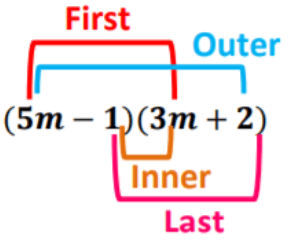Explain to students that to avoid confusion, they can think of the binomials as two lists of two terms, each with a particular sign. Encourage the students not to think about operations in their answer, but just list each term with its sign. For instance (5m, -1) and (3m, 2).

It is useful to present the operations with each term in brackets from here.

In other words:

(5m – 1)(3m + 2) = (5m)(3m) + (5m)(2) + (-1)(3m) + (-1)(2) =

15m² + 10m + (-3m) + (-2) =

15m²+ 10m – 3m – 2 =

15m² + 7m – 2

## Activities to Practice Multiplying Binomials

### Quiz

To implement this quiz activity in your classroom, you’ll need to prepare a PowerPoint with different binomial multiplication problems. Each slide contains one binomial problem that students have to solve in a given timeframe (for example, you can choose to give 5 minutes per problem).

You can use the binomial problems from this Exit Quiz Worksheet (Members Only) or from our free Assignment Worksheet. Make sure to also bring a timer (or alternatively, rely on your phone) to measure the time for a given problem.

Divide students into groups of 3, 4 and start playing! The group that has the most correct answers, in the end, wins the game. You can also introduce a symbolic prize for the quiz winners, such as a ‘no homework for a week’ prize!

If you’re a parent that’s homeschooling their child or children, you can easily adapt this activity by using the mentioned worksheets for an individual activity. Alternatively, if you have an older child as well, have them join in the quiz and add plenty of candy as prizes for the winner!

## Before You Leave…

If you enjoyed the tips in this article, you’ll definitely love our lesson that goes in depth in teaching kids how to multiply binomials!

So if you’re looking for more math materials for children of all ages, sign up for our emails for plenty of free lessons, worksheets, bell-work, and much more! Feel free to also head over to our blog.

And if you’d like to become a member, sign up at Math Teacher Coach!